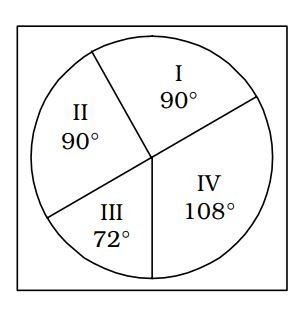## Introduction to Data Interpretation

#### Data Interpretation

Direction: The following pie-chart shows the percentage distribution of the expenditure incurred in publishing a book. Read the pie-chart and answer the questions.1. The central angle of the sector corresponding to the expenditure incurred on Royalty is :

1. On the basis of given pie chart in question ,
Percentage expense on royalty = 15%
Percent of total expenditure = 100%
Corresponding angle for total expenditure = 360°
∵ 100% ≡ 360°

 ∴ Required angle = Corresponding angle for total expenditure × Percentage expense on royalty = 54° Percent of total expenditure

##### Correct Option: C

On the basis of given pie chart in question ,
Percentage expense on royalty = 15%
Percent of total expenditure = 100%
Corresponding angle for total expenditure = 360°
∵ 100% ≡ 360°

 ∴ Required angle = Corresponding angle for total expenditure × Percentage expense on royalty = 54° Percent of total expenditure

 ∴ Required angle = 360 × 15 = 54° 100

1. Royalty on the book is less than the printing cost by :

1. According to given pie chart in question ,
Percent of royalty cost = 20%
Percent of printing cost = 15%
Change in cost = 20 - 15 = 5%

 Required percent =Change in cost × 100% Percent of royalty cost

##### Correct Option: C

According to given pie chart in question ,
Percent of royalty cost = 20%
Percent of printing cost = 15%
Change in cost = 20 - 15 = 5%

 Required percent =Change in cost × 100% Percent of royalty cost

 Required percent =20 - 15 × 100% 20

 Required percent =5 × 100% = 25% 20

Direction: Study the pie chart and answer the given questions. The total expenditure of a company for a particular month is Rs. 60000. The various heads of expenditure I to IV are indicated in a pie chart given below. These heads are :
I. Raw materials
II. Conveyance
III. Electricity1. What percentage of total expenditure is on raw materials?

1. On the basis of given pie chart in question ,
Corresponding angle for raw materials = 90°
Percent of total expenditure = 100%
Corresponding angle for total expenditure = 360°
we know that , 360° ≡ 100%

##### Correct Option: A

On the basis of given pie chart in question ,
Corresponding angle for raw materials = 90°
Percent of total expenditure = 100%
Corresponding angle for total expenditure = 360°
we know that , 360° ≡ 100%

 ∴ Required percent = 90° ≡ 100 × 90 = 25% 360

1. What is the amount spent on overhead expenses?

1. According to given pie chart in question ,
Corresponding angle for overhead expenses = 108°
Total expenditure = \$ 60000
⇒ 360° ≡ \$ 60000

 ∴ Required amount = Total expenditure × Corresponding angle for overhead expenses Total angle for expenditure

##### Correct Option: C

According to given pie chart in question ,
Corresponding angle for overhead expenses = 108°
Total expenditure = \$ 60000
⇒ 360° ≡ \$ 60000

 ∴ Required amount = Total expenditure × Corresponding angle for overhead expenses Total angle for expenditure

 ∴ Required amount = 108° ≡ 60000 × 108 = \$ 18000 360

1. Total expenditure on conveyance is :

1. As per the given pie chart in question ,
Corresponding angle for conveyance = 90°
Total expenditure = 360° ≡ \$ 60000

 ∴ Total expenditure on conveyance = 90° ≡ Total expenditure × Corresponding angle for conveyance 360

##### Correct Option: B

As per the given pie chart in question ,
Corresponding angle for conveyance = 90°
Total expenditure = 360° ≡ \$ 60000

 ∴ Total expenditure on conveyance = 90° ≡ Total expenditure × Corresponding angle for conveyance 360

 Total expenditure on conveyance = 60000 × 90 = \$ 15000 360